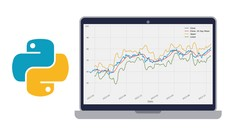# Python for Financial Analysis and Algorithmic TradingOnline Courses Udemy - Python for Financial Analysis and Algorithmic Trading, Learn numpy , pandas , matplotlib , quantopian , finance , and more for algorithmic trading with Python!

BESTSELLER, 4.5 (8,446 ratings), Created by Jose Portilla, English [Auto-generated], French [Auto-generated], 8 more

PREVIEW THIS COURSE -.> GET COUPON CODE

What you'll learn

• Use NumPy to quickly work with Numerical Data
• Use Pandas for Analyze and Visualize Data
• Use Matplotlib to create custom plots
• Learn how to use statsmodels for Time Series Analysis
• Calculate Financial Statistics, such as Daily Returns, Cumulative Returns, Volatility, etc..
• Use Exponentially Weighted Moving Averages
• Use ARIMA models on Time Series Data
• Calculate the Sharpe Ratio
• Optimize Portfolio Allocations
• Understand the Capital Asset Pricing Model
• Learn about the Efficient Market Hypothesis
• Conduct algorithmic Trading on Quantopian